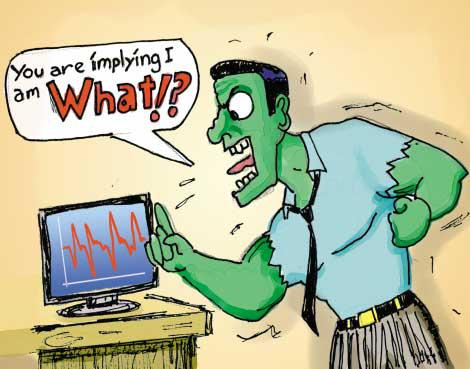# What is Implied by Implied Volatility?

## Elie Ayache takes his hammer to some cherished conceptsWord and concept

Implied volatility is not just a word or a concept. As a word, what is implied by implied volatility – what “implied volatility” means – is the value of the Brownian diffusion coefficient, inherent in the Black-Scholes theory, that you get by inverting the Black-Scholes formula for the theoretical value of a European option against the empirical market price of that option. From which you immediately see that the meaning of the word “implied volatility,” as is always the case with meaning, will depend on the meaning, I dare even say the meaningfulness, the validity, of other group of words, perhaps theories or even whole conceptual schemes, in this case “Black-Scholes.” We shall spend time later exploring the exact meaning of this – what this means exactly that, in order to fulfill the meaning of “implied volatility,”one should make sure that the Black-Scholes formula validly implies it. It will all hinge, as you will see, on the notion of hedge. The Black-Scholes formula reposes on the continuous hedging argument. Therefore you have to first make sure that the hedging is validly conceived in order to guarantee that the Black-Scholes formula may imply something like “implied volatility.” Continuous hedging is thus part of the meaning of implied volatility, not to say its presupposition. Consequently your next concern, after implying implied volatility, ought to be: “How was the hedging achieved already?” Or more specifically: “Under what volatility was the hedging achieved?” This question is all the more pressing that, by the very meaning of “implied volatility,” there is a question mark hanging over the value of volatility.

At this point, all this may sound trivial and tautological to you, for isn’t the meaning of “volatility” in Black-Scholes precisely the number that you should use in the formula, both for the pricing and the hedging? And could therefore be any question that this number should be used in the algorithm to compute the hedge and no other? Actually, the answer is far from clear, for you might legitimately wonder, given that hedging is not just the formal argument used in the formal derivation of Black-Scholes but has, among other material consequences, well, hedging, and the corresponding course of action that will unfold in time, whether real volatility will actually turn out to be the same as implied. For surely we don’t live in the world described by Black-Scholes; and this is no accident, for isn’t the true purpose of option trading, consequently of “implied volatility,” to detect discrepancies in the market prices of options and try to make some money by buying or selling these options; that is to say, by arbitraging their prices against what will turn out to be their “true value”? And isn’t this true value the reflection of the cost of the actual dynamic hedging that will ensue?

What we are witnessing here are the implications of “implied volatility” as word quickly developing into the implications of “implied volatility” as concept. While “implied volatility” as a word implied hedging under implied volatility – and no other number – by the simple logic, or meaning, of Black-Scholes (it implied it analytically, in philosophical parlance), “implied volatility” as a concept implies a lot more. With the concept of implied volatility, we step beyond the analysis of the Black-Scholes formula and the success or failure of inverting it. We question the validity, the  significance, and the implications of such an act. We wonder what it may mean for the whole intellectual landscape lying behind the formula, and the whole scientific practice that is conditioned by it, that the number called “volatility” should be retrieved from the prices of options traded in something called the “market.”

What I am suggesting here is that “implied volatility” is mainly a trading concept. For instance it can be taught in an option trading school and we can try and picture the consequences of such a teaching and such a program, i.e. the subsequent rule of action, the subsequent disciplined behavior. As a trading concept, implied volatility says: “From now on, you shall go and find in the option market itself, the answer to the question about that unobservable parameter that goes in the Black-Scholes equation.” A lot is involved in that single step, not least among which the admission that the market may indeed act as an independent physical reality that we may confront with our theory and calibrate our theoretical model against.